257
Sorting - Code Monk
Sorting
Sorting-algorithm
CodeMonk

Sorting is a process of arranging items in ascending or descending order. This process can be implemented via many different algorithms.

Following is the list of sorting algorithms which will be explained in this tutorial:

• Bubble Sort
• Selection Sort
• Insertion Sort
• Merge Sort
• Quicksort
• Count Sort

Bubble Sort: This algorithm is based on the idea of repeatedly comparing pairs of adjacent elements and then switching their positions if they exist in the wrong order. The pseudo-code is given below

Let A [ ] is an unsorted array of n elements. We need it to be sorted in increasing order .

  void bubble_sort( int A[ ], int n ) {
int temp;
for(int k = 0; k< n-1; k++) {
// (n-k-1) is for ignoring comparisons of elements which have already been compared in earlier iterations

for(int i = 0; i < n-k-1; i++) {
if(A[ i ] > A[ i+1] ) {
// here swapping of positions is being done.
temp = A[ i ];
A[ i ] = A[ i+1 ];
A[ i + 1] = temp ;
}
}
}
}


Lets try to understand above code with an example: A [ ] = { 7, 4, 5, 2}.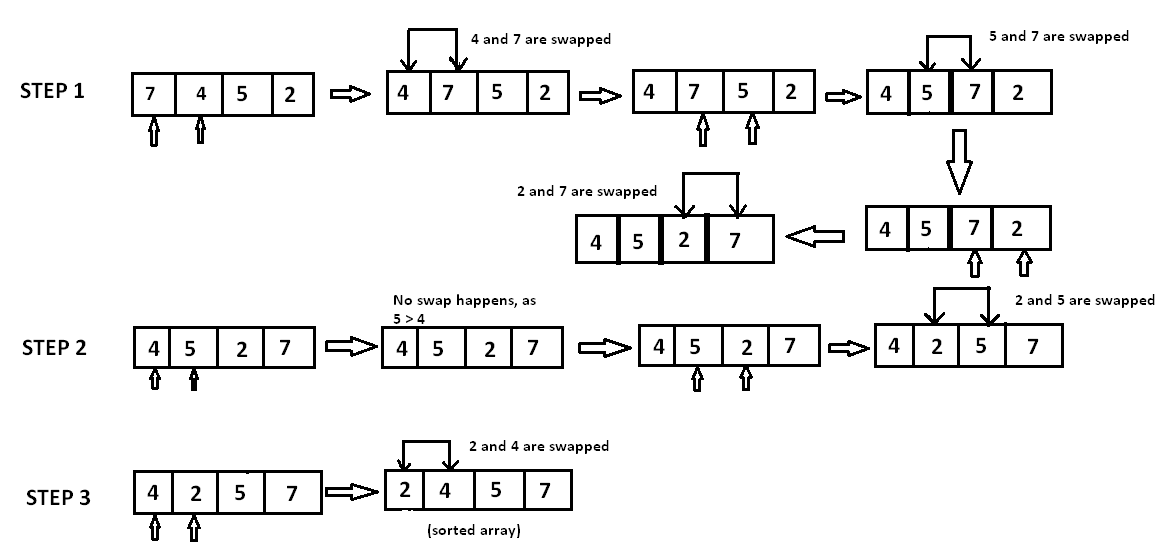In the first step, the first element 7 is compared with 4 and since 7 is greater than 4 both are swapped. Then, 7 is compared with 5 and swapped and so on. As all the elements are smaller than 7, therefore 7 is eventually placed at last position of the array.

Similarly, in the second step, we start with the first element of array i.e., 4 and then compare it with 5, as 5 is greater than 4, no swap happens. When 5 is compared with 2, they are swapped. Elements which have been iterated on are not evaluated in subsequent iterations.

In third step, we compare 4 with 2 and swap - and finally, we get a sorted array.

Complexity: The complexity of bubble sort is O(n2) in the worst and average case because for every element we iterate over the the entire array each time.

Selection Sort: This algorithm is based on the idea of finding the minimum or maximum element in the unsorted array and then putting it in its correct position for a sorted array.

We have an array A [ ] = {7, 5, 4, 2} and we need to sort it in ascending order.

Let’s find the minimum element in the array i.e., 2 and then replace it with the first position's element, i.e., 7. Now we find the second largest element in the remaining unsorted array and put it at the second position and so on.

Let’s take a look at the implementation.

Here n is the number of elements in the array.

    void selection_sort (int A[ ], int n) {
// temporary variable to store the position of minimum element

int minimum;
// reduces the effective size of the array by one in  each iteration.

for(int i = 0; i < n-1 ; i++)  {

// assuming first element to be minimum of the  unsorted array .
minimum = i ;

// gives the effective size of unsorted  array .

for(int j = i+1; j < n ; j++ ) {
if(A[ j ] < A[ minimum ])  {                //finds the minimum element
minimum = j ;
}
}
// putting minimum element on its proper position.
swap ( A[ minimum ], A[ i ]) ;
}
}


At ith iteration,elements from position 0 to i-1 ,will be sorted.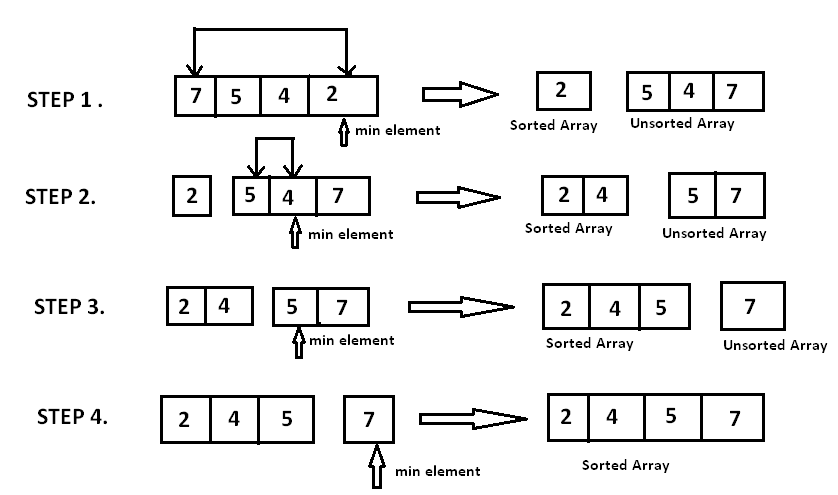Complexity : Here as to find the minimum element from the array of n elements, we require n-1 comparisons to be performed. Then, after putting minimum element to its proper position, size of unsorted array reduces to n-1 and then n-2 comparisons are required to find the minimum in the unsorted array. Therefore (n-1) + (n-2 ) + .......+ 1 = ( n * (n-1) ) / 2 comparisons and n exchanges (swapping ), which gives the complexity of O( n2 ).

Insertion Sort: The idea behind is that in each iteration, it consumes one element from the input elements, removes it and finds its correct position i.e., where it belongs in the sorted list and places it there.

It iterates the array by growing the sorted list behind it at each iteration. It checks the current element with the largest value in the sorted list. If the current element is larger, then it leaves the element at its place and moves to the next element else it finds its correct position in the sorted list and moves it to that position. It is done by shifting all the elements which are larger than the current element to one position ahead.

Implementation:

void insertion_sort ( int A[ ] , int n) {
for( int i = 0 ;i < n ; i++ ) {
/*storing current element whose left side is checked for its
correct position .*/

int temp = A[ i ];
int j = i;

/* check whether the adjacent element in left side is greater or
less than the current element. */

while( temp < A[ j -1] && j > 0 ) {

// moving the left side element to one position forward .
A[ j ] = A[ j-1];
j= j - 1;

}
// moving current element to its  correct position.
A[ j ] = temp;
}
}


Above code will be more clear with pictorial representation of the example: Take array A[ ] = {7, 4, 5, 2} .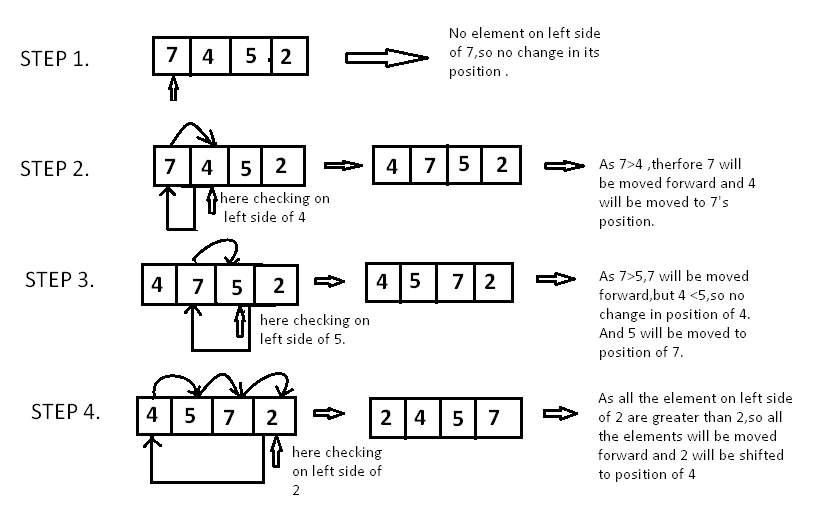Since, 7 as the first element has no other element to be compared with, it remains at its position. Now when we move towards 4, we have 7 which is the largest element in the sorted list and greater than 4. So we will move 4 to its correct position. Similarly with 5, as 7 (largest element in the sorted list) is greater than 5, we will move 5 to its correct position. Finally for 2, all the elements on left side of 2 (sorted list) are moved one position forward as all are greater than 2 and then 2 is placed on first position. Finally you will get a sorted array.

Complexity : Complexity of Insertion sort is O(n2) .

Merge Sort: This sorting algorithm works on the following principle - Divide the array in two halves. Repeatedly sort each half, then merge two halves.

Lets say we have an array A[ ] = { 9, 7, 8, 3, 2, 1} .

First we will divide it in two halves A1 [ ] = {9, 7, 8} and A2[ ] = {3, 2, 1}. Again divide these 2 halves in their two halves. For A1 it will be A1_a[ ] = {9, 7} and A1_b[ ] = {8}. Again, divide A1_a and then as they further cannot be divided, so merge them by comparing them. A1_a will be {7, 9} and then compare and merge A1_a and A1_b. Now, A1 will be { 7, 8, 9} . Do same for A2 and then A2 will be {1, 2, 3} . Now, compare A1 and A2 and then merge them. Now, A will be {1, 2, 3, 7, 8, 9}.

Let’s implement this:

First we will see how we can merge the 2 arrays by comparing them. A[ ] - array whose elements are to be sorted. start – starting position of an array . end – ending position of an array. mid – middle position of array .

We will take an auxiliary array say Arr[ ], which is used to store the sorted version of the real array.

void merge(int A[ ] , int start, int mid, int end) {

//stores the starting position of both parts in temporary variables.
int p = start ,q = mid+1;

int Arr[end-start+1] , k=0;

for(int i = start ;i <= end ;i++) {
if(p > mid)      //checks if first part comes to an end or not .
Arr[ k++ ] = A[ q++] ;

else if ( q > end)   //checks if second part comes to an end or not
Arr[ k++ ] = A[ p++ ];

else if( A[ p ] < A[ q ])     //checks which part has smaller element.
Arr[ k++ ] = A[ p++ ];

else
Arr[ k++ ] = A[ q++];
}
for (int p=0 ; p< k ;p ++) {
/* Now the real array has elements in sorted manner including both
parts.*/
A[ start++ ] = Arr[ p ] ;
}
}


Here in merge function, we will merge the two part of arrays where one part has starting and ending positions from start to mid respectively and another part has positions from mid+1 to end.

We will start from starting positions of both the parts that are p and q.Then we will compare respective elements of both the parts and the one with the smaller value will be stored in the auxiliary array (Arr[ ]). If at some condition ,one part comes to end ,then all the elements of another part of array are added in the auxiliary array in the same order they exist.

Now lets come to 2 branched recursive function :

void merge_sort (int A[ ] , int start , int end ) {
if( start < end ) {
int mid = (start + end ) / 2 ;           // defines the current array in 2 parts .
merge_sort (A, start , mid ) ;                 // sort the 1st part of array .
merge_sort (A,mid+1 , end ) ;              // sort the 2nd part of array.

// merge the both parts by comparing elements of both the parts.
merge(A,start , mid , end );
}
}


This will be more clear with the pictorial representation of the above explained example.

We you can see the order of the recursive calls. After 3rd and 4th recursive call, no further recursive calls can take place, so they are merged in the 5th function call of merge function.

Similarly after 5th and 6th call, elements are merged by 7th function call of merge function. Similarly after 10th and 11th call, elements are merged into 12th function call of merge function. Similarly after 12th and 13th call, elements are merged in 14th function call of merge function. Finally elements after 7th and 14th call are merged in 15th call of merge function and you get a sorted array.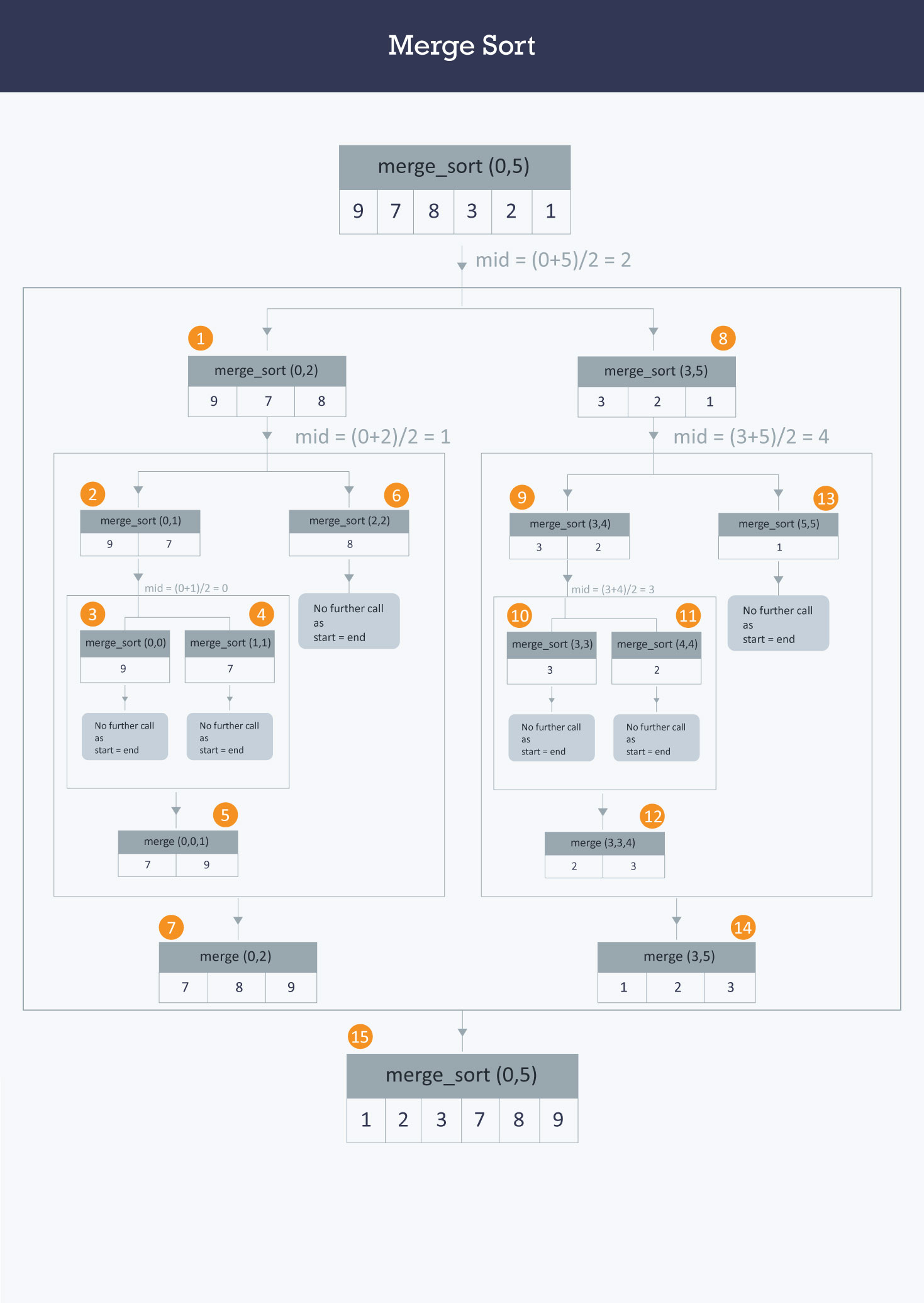Complexity: Array with n elements is divided recursively in 2 parts, so it will form a tree with nodes as divided parts of array ( subproblems ). The height of the tree will be log2n and at each level of tree the computation cost of all the subproblems will be n. At each level the merge operation will take O( n ) time. So the overall complexity of this algorithm will be O( n( log2 n )).

Quick Sort: This algorithm is also based on the divide and conquer approach. It reduces the space complexity and removes the use of auxiliary array used in merge sort.

Idea: It is based on the idea of choosing one element as pivot element and partitioning the array around it such that the left side of pivot contains all elements less than the pivot element and right side contains all elements greater than the pivot.

Selecting a random pivot in an array results into an improved time complexity in average cases.

Implementation:
Choose the first element of array as pivot element First, we will see how the partition of the array takes place around the pivot.

Idea: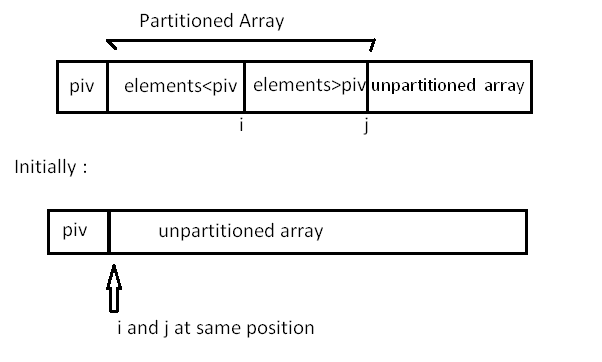Here, A[ ] = array whose elements are to be sorted.
start = leftmost position of the array.
end = rightmost position of the array.
i = boundary point between those less than pivot and those greater than pivot .
j = boundary point between partitioned and unpartitioned part of array.
piv = pivot element .

int partition ( int A[],int start ,int end) {
int i = start + 1;
int piv = A[start] ;            //make the first element as pivot element.
for(int j =start + 1; j <= end ; j++ )  {
/*rearrange the array by putting elements which are less than pivot
on one side and which are greater that on other. */

if ( A[ j ] < piv) {
swap (A[ i ],A [ j ]);
i += 1;
}
}
swap ( A[ start ] ,A[ i-1 ] ) ;  //put the pivot element in its proper place.
return i-1;                      //return the position of the pivot
}


Now, let us see the recursive function Quick_sort :

void quick_sort ( int A[ ] ,int start , int end ) {
if( start < end ) {
//stores the position of pivot element
int piv_pos = partition (A,start , end ) ;
quick_sort (A,start , piv_pos -1);    //sorts the left side of pivot.
quick_sort ( A,piv_pos +1 , end) ; //sorts the right side of pivot.
}
}


Here we find the proper position of the pivot element by rearranging the array using partition function. Then we divide the array into two halves left side of the pivot(Elements less than pivot element) and right side of the pivot (elements greater than pivot element) and apply the same step recursively.

Example:
You have an array A[ ] = { 9, 7, 8, 3, 2, 1} .
Below you can see ,that rand_partition function chooses pivot randomly as 7 and then swap it with first element of array and then partition function call takes place,which divides the array in two halves. First half has elements less than 7 and other has greater than 7 .
For elements less than 7,in 5th call,rand_partition function chooses 2 as pivot element randomly and then swap it with first element and call to partition function takes place. After 7thand 8th call ,no further calls can takes place as only one element left in both the calls.
Similarly you can observe the order of calls for the elements greater than 7 .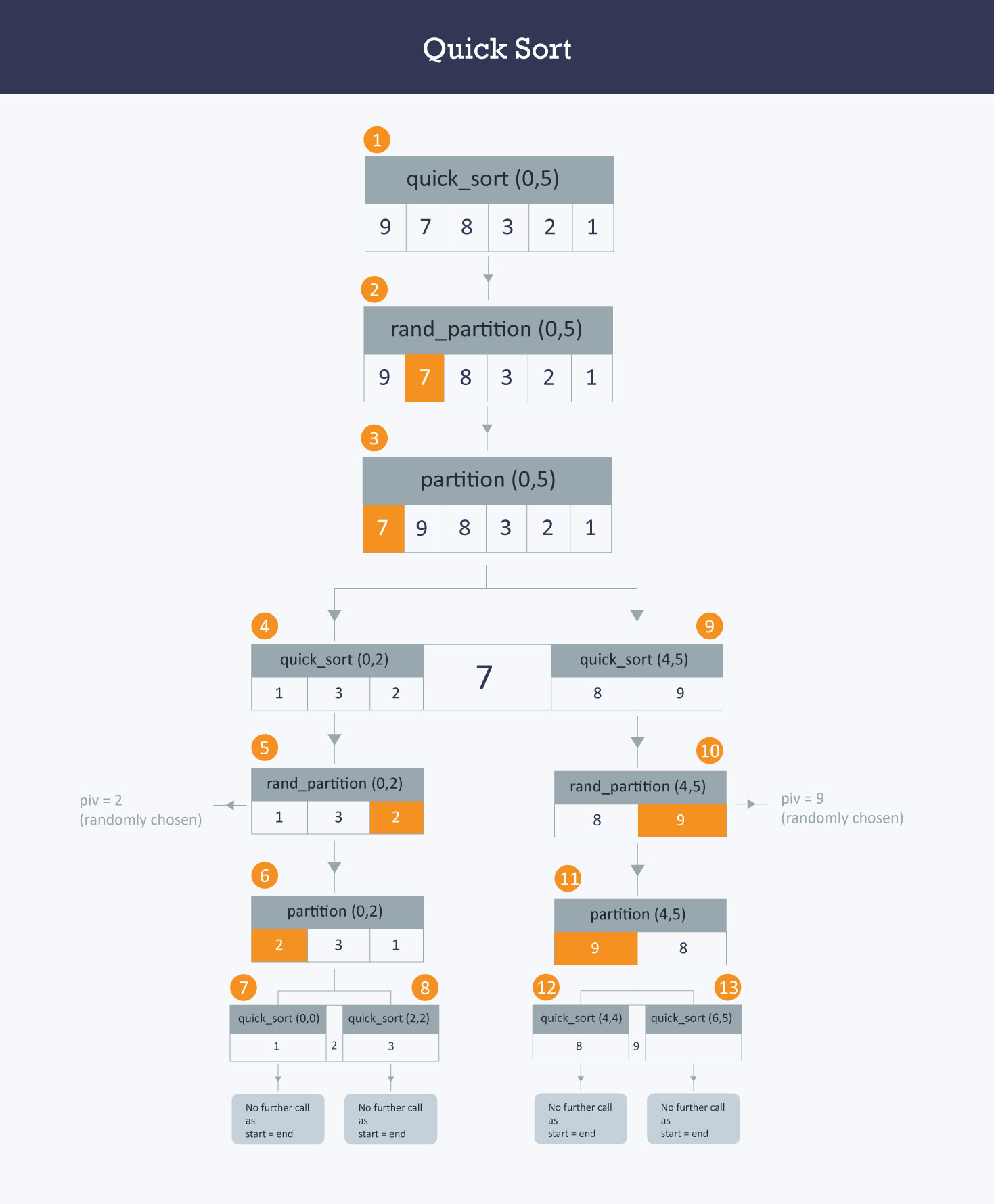Let’s see the randomized version of the partition function :

int rand_partition ( int A[ ] , int start , int end ) {
//chooses position of pivot randomly by using rand() function .
int random = start + rand( )%(end-start +1 ) ;

swap ( A[random] , A[start]) ;        //swap pivot with 1st element.
return partition(A,start ,end) ;       //call the above partition func.
}


Use rand_partition instead of partition function in quick_sort function to reduce the time complexity of this algorithm.

Complexity: The worst case time complexity of this algorithm is O( n2 ), but as this is randomized algorithm, its time complexity fluctuates between O( n2 ) and O(n (log n) ) and mostly it comes out to be O( n (log n)) .

Counting Sort: In this sort, we count the frequencies of distinct elements of array and store them in an auxiliary array, by mapping its value as index of auxiliary array and then place each element in its proper position in the output array .

Implementation :

Let the maximum element which can be in the array is max1 .
Now take an array having size of max1 +1 .Let it be Aux[0 ...max1] .
A[ ] = array whose elements are to be sorted (n elements ).
Out[ ] = array having sorted version of A[ ] .

void counting_sort (int A[ ],int Aux[ ] ,int Out[ ],int n ) {
// initialize the elements of array with 0 .
for(int i = 0 ; i <= max1 ; i ++ )
Aux[ i ] = 0 ;

/*stores the frequencies of each distinct element of A[ ],by
mapping its value as the index of Aux[ ] array.*/
for( int j = 0; j < n ;j++ )
Aux[ A [ j ] ] ++ ;

/*Calculates how many elements are less than or equal to i by
running sum of  Aux array. */
for(int i = 1; i <= max1 ;i++ )
Aux [ i ] = Aux[ i ] + Aux [ i-1 ];
//places each element of A[ ] at its correct position int Out [ ] array.
for (int j = n-1 ; j >= 0 ; j--)
{
Out[ Aux[ A[ j ] ]-1] = A[ j ];
Aux[A[ j ] ] = Aux[ A[ j ]] -1;
}
}


Lets try to understand it with an example :

Let A[ ] be { 4 , 5 , 4 , 3 , 4 ,2 , 1 ,6 , 1} and value of max1 be 7 .
Then: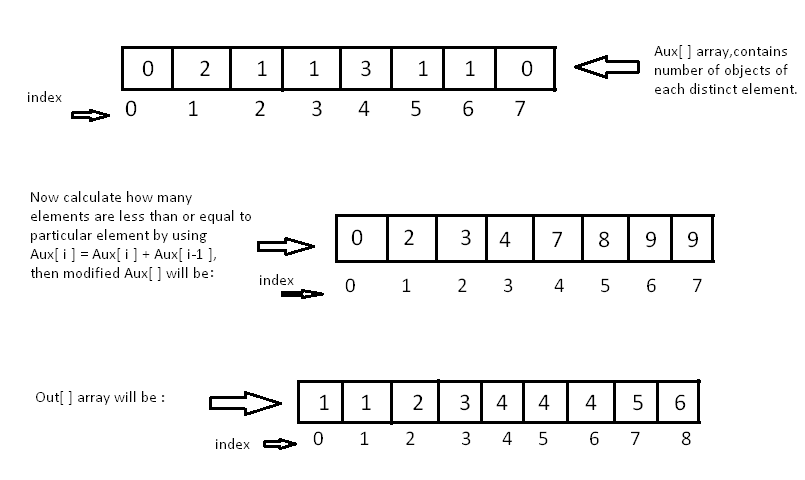Complexity: As the above code runs in linear time so the complexity in worst case will be O(max + n), where n is the number of elements and max is the range of input element of array A[ ].